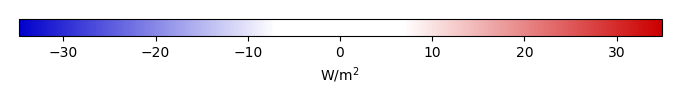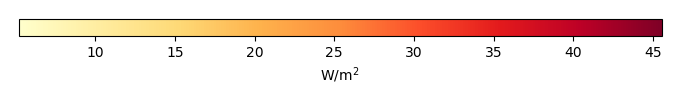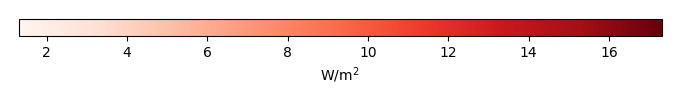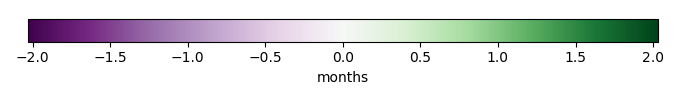# Mean State

Period Mean (original grids) [W/m2]
Model Period Mean (intersection) [W/m2]
Model Period Mean (complement) [W/m2]
Benchmark Period Mean (intersection) [W/m2]
Benchmark Period Mean (complement) [W/m2]
Bias [W/m2]
RMSE [W/m2]
Phase Shift [months]
Bias Score 
RMSE Score 
Seasonal Cycle Score 
Spatial Distribution Score 
Interannual Variability Score 
Overall Score 
Benchmark [-] 187.
CLM5PHSOFF [-] 181. 181. 186. 206. -4.89 15.2 0.808 0.720 0.495 0.903 0.940 0.800 0.726
CLM5PHSON [-] 182. 182. 186. 206. -4.45 15.1 0.823 0.728 0.493 0.901 0.939 0.801 0.726
Period Mean (original grids) [W/m2]
Model Period Mean (intersection) [W/m2]
Model Period Mean (complement) [W/m2]
Benchmark Period Mean (intersection) [W/m2]
Benchmark Period Mean (complement) [W/m2]
Bias [W/m2]
RMSE [W/m2]
Phase Shift [months]
Bias Score 
RMSE Score 
Seasonal Cycle Score 
Spatial Distribution Score 
Interannual Variability Score 
Overall Score 
Benchmark [-] 198.
CLM5PHSOFF [-] 194. 194. 198. 214. -3.94 14.6 0.751 0.704 0.510 0.913 0.974 0.850 0.743
CLM5PHSON [-] 195. 195. 198. 214. -2.80 14.2 0.767 0.714 0.516 0.906 0.964 0.852 0.745
Period Mean (original grids) [W/m2]
Model Period Mean (intersection) [W/m2]
Model Period Mean (complement) [W/m2]
Benchmark Period Mean (intersection) [W/m2]
Benchmark Period Mean (complement) [W/m2]
Bias [W/m2]
RMSE [W/m2]
Phase Shift [months]
Bias Score 
RMSE Score 
Seasonal Cycle Score 
Spatial Distribution Score 
Interannual Variability Score 
Overall Score 
Benchmark [-] 199.
CLM5PHSOFF [-] 195. 195. 198. 219. -3.15 15.5 0.706 0.795 0.573 0.904 0.994 0.748 0.765
CLM5PHSON [-] 196. 196. 198. 219. -2.87 15.6 0.787 0.797 0.569 0.893 0.996 0.748 0.762
Period Mean (original grids) [W/m2]
Model Period Mean (intersection) [W/m2]
Model Period Mean (complement) [W/m2]
Benchmark Period Mean (intersection) [W/m2]
Benchmark Period Mean (complement) [W/m2]
Bias [W/m2]
RMSE [W/m2]
Phase Shift [months]
Bias Score 
RMSE Score 
Seasonal Cycle Score 
Spatial Distribution Score 
Interannual Variability Score 
Overall Score 
Benchmark [-] 70.7
CLM5PHSOFF [-] 69.4 69.6 70.5 72.8 -0.883 18.9 0.310 0.906 0.766 0.979 0.996 0.737 0.858
CLM5PHSON [-] 73.1 73.3 70.5 72.8 1.86 18.4 0.331 0.906 0.772 0.978 0.996 0.741 0.861
Period Mean (original grids) [W/m2]
Model Period Mean (intersection) [W/m2]
Model Period Mean (complement) [W/m2]
Benchmark Period Mean (intersection) [W/m2]
Benchmark Period Mean (complement) [W/m2]
Bias [W/m2]
RMSE [W/m2]
Phase Shift [months]
Bias Score 
RMSE Score 
Seasonal Cycle Score 
Spatial Distribution Score 
Interannual Variability Score 
Overall Score 
Benchmark [-] 194.
CLM5PHSOFF [-] 179. 178. 190. 207. -11.6 19.3 1.25 0.532 0.437 0.803 0.957 0.769 0.656
CLM5PHSON [-] 175. 175. 190. 207. -11.2 19.1 1.25 0.542 0.437 0.802 0.963 0.769 0.658
Period Mean (original grids) [W/m2]
Model Period Mean (intersection) [W/m2]
Model Period Mean (complement) [W/m2]
Benchmark Period Mean (intersection) [W/m2]
Benchmark Period Mean (complement) [W/m2]
Bias [W/m2]
RMSE [W/m2]
Phase Shift [months]
Bias Score 
RMSE Score 
Seasonal Cycle Score 
Spatial Distribution Score 
Interannual Variability Score 
Overall Score 
Benchmark [-] 97.8
CLM5PHSOFF [-] 96.3 96.2 97.6 118. -1.32 17.7 0.230 0.919 0.787 0.985 0.987 0.707 0.862
CLM5PHSON [-] 100. 100. 97.6 118. 2.99 15.5 0.231 0.921 0.817 0.985 0.995 0.687 0.870
Period Mean (original grids) [W/m2]
Model Period Mean (intersection) [W/m2]
Model Period Mean (complement) [W/m2]
Benchmark Period Mean (intersection) [W/m2]
Benchmark Period Mean (complement) [W/m2]
Bias [W/m2]
RMSE [W/m2]
Phase Shift [months]
Bias Score 
RMSE Score 
Seasonal Cycle Score 
Spatial Distribution Score 
Interannual Variability Score 
Overall Score 
Benchmark [-] 139.
CLM5PHSOFF [-] 135. 135. 138. 149. -3.17 16.1 0.353 0.880 0.764 0.970 0.999 0.671 0.842
CLM5PHSON [-] 135. 135. 138. 149. -2.61 15.9 0.340 0.883 0.767 0.972 0.999 0.666 0.842
Period Mean (original grids) [W/m2]
Model Period Mean (intersection) [W/m2]
Model Period Mean (complement) [W/m2]
Benchmark Period Mean (intersection) [W/m2]
Benchmark Period Mean (complement) [W/m2]
Bias [W/m2]
RMSE [W/m2]
Phase Shift [months]
Bias Score 
RMSE Score 
Seasonal Cycle Score 
Spatial Distribution Score 
Interannual Variability Score 
Overall Score 
Benchmark [-] 181.
CLM5PHSOFF [-] 175. 175. 179. 205. -4.15 18.2 0.328 0.763 0.611 0.974 0.938 0.719 0.769
CLM5PHSON [-] 174. 174. 179. 205. -3.72 18.1 0.320 0.770 0.610 0.974 0.938 0.722 0.771
Period Mean (original grids) [W/m2]
Model Period Mean (intersection) [W/m2]
Model Period Mean (complement) [W/m2]
Benchmark Period Mean (intersection) [W/m2]
Benchmark Period Mean (complement) [W/m2]
Bias [W/m2]
RMSE [W/m2]
Phase Shift [months]
Bias Score 
RMSE Score 
Seasonal Cycle Score 
Spatial Distribution Score 
Interannual Variability Score 
Overall Score 
Benchmark [-] 62.8
CLM5PHSOFF [-] 62.7 63.1 63.2 54.4 -0.0956 15.9 0.179 0.929 0.788 0.988 0.991 0.738 0.870
CLM5PHSON [-] 64.7 65.0 63.2 54.4 0.684 15.6 0.199 0.931 0.792 0.987 0.990 0.748 0.873
Period Mean (original grids) [W/m2]
Model Period Mean (intersection) [W/m2]
Model Period Mean (complement) [W/m2]
Benchmark Period Mean (intersection) [W/m2]
Benchmark Period Mean (complement) [W/m2]
Bias [W/m2]
RMSE [W/m2]
Phase Shift [months]
Bias Score 
RMSE Score 
Seasonal Cycle Score 
Spatial Distribution Score 
Interannual Variability Score 
Overall Score 
Benchmark [-] 198.
CLM5PHSOFF [-] 190. 190. 198. 197. -8.29 16.0 0.373 0.833 0.763 0.972 1.00 0.713 0.841
CLM5PHSON [-] 192. 192. 198. 197. -7.59 15.6 0.385 0.846 0.765 0.971 0.999 0.718 0.844
Period Mean (original grids) [W/m2]
Model Period Mean (intersection) [W/m2]
Model Period Mean (complement) [W/m2]
Benchmark Period Mean (intersection) [W/m2]
Benchmark Period Mean (complement) [W/m2]
Bias [W/m2]
RMSE [W/m2]
Phase Shift [months]
Bias Score 
RMSE Score 
Seasonal Cycle Score 
Spatial Distribution Score 
Interannual Variability Score 
Overall Score 
Benchmark [-] 120.
CLM5PHSOFF [-] 115. 115. 118. 145. -2.77 13.7 0.238 0.917 0.840 0.984 0.991 0.664 0.873
CLM5PHSON [-] 113. 113. 118. 145. -2.30 13.6 0.227 0.921 0.839 0.985 0.990 0.660 0.872
Period Mean (original grids) [W/m2]
Model Period Mean (intersection) [W/m2]
Model Period Mean (complement) [W/m2]
Benchmark Period Mean (intersection) [W/m2]
Benchmark Period Mean (complement) [W/m2]
Bias [W/m2]
RMSE [W/m2]
Phase Shift [months]
Bias Score 
RMSE Score 
Seasonal Cycle Score 
Spatial Distribution Score 
Interannual Variability Score 
Overall Score 
Benchmark [-] 163.
CLM5PHSOFF [-] 136. 136. 142. 173. -5.43 16.4 0.384 0.813 0.701 0.960 0.998 0.716 0.815
CLM5PHSON [-] 137. 137. 142. 173. -4.59 16.0 0.394 0.818 0.705 0.958 0.998 0.713 0.816
Period Mean (original grids) [W/m2]
Model Period Mean (intersection) [W/m2]
Model Period Mean (complement) [W/m2]
Benchmark Period Mean (intersection) [W/m2]
Benchmark Period Mean (complement) [W/m2]
Bias [W/m2]
RMSE [W/m2]
Phase Shift [months]
Bias Score 
RMSE Score 
Seasonal Cycle Score 
Spatial Distribution Score 
Interannual Variability Score 
Overall Score 
Benchmark [-] 177.
CLM5PHSOFF [-] 163. 163. 175. 217. -12.2 17.9 0.296 0.707 0.763 0.978 0.963 0.676 0.808
CLM5PHSON [-] 162. 162. 175. 217. -12.0 18.0 0.296 0.708 0.763 0.978 0.960 0.674 0.808
Period Mean (original grids) [W/m2]
Model Period Mean (intersection) [W/m2]
Model Period Mean (complement) [W/m2]
Benchmark Period Mean (intersection) [W/m2]
Benchmark Period Mean (complement) [W/m2]
Bias [W/m2]
RMSE [W/m2]
Phase Shift [months]
Bias Score 
RMSE Score 
Seasonal Cycle Score 
Spatial Distribution Score 
Interannual Variability Score 
Overall Score 
Benchmark [-] 69.7
CLM5PHSOFF [-] 68.1 67.9 69.6 71.1 -1.70 17.5 0.0891 0.916 0.779 0.994 0.988 0.750 0.868
CLM5PHSON [-] 70.7 70.6 69.6 71.1 -0.616 17.1 0.0938 0.915 0.784 0.994 0.981 0.747 0.867
Period Mean (original grids) [W/m2]
Model Period Mean (intersection) [W/m2]
Model Period Mean (complement) [W/m2]
Benchmark Period Mean (intersection) [W/m2]
Benchmark Period Mean (complement) [W/m2]
Bias [W/m2]
RMSE [W/m2]
Phase Shift [months]
Bias Score 
RMSE Score 
Seasonal Cycle Score 
Spatial Distribution Score 
Interannual Variability Score 
Overall Score 
Benchmark [-] 204.
CLM5PHSOFF [-] 193. 193. 201. 219. -7.37 17.0 0.480 0.760 0.678 0.943 0.988 0.751 0.800
CLM5PHSON [-] 192. 192. 201. 219. -6.91 16.8 0.470 0.768 0.678 0.943 0.986 0.754 0.801
Period Mean (original grids) [W/m2]
Model Period Mean (intersection) [W/m2]
Model Period Mean (complement) [W/m2]
Benchmark Period Mean (intersection) [W/m2]
Benchmark Period Mean (complement) [W/m2]
Bias [W/m2]
RMSE [W/m2]
Phase Shift [months]
Bias Score 
RMSE Score 
Seasonal Cycle Score 
Spatial Distribution Score 
Interannual Variability Score 
Overall Score 
Benchmark [-] 150.
CLM5PHSOFF [-] 145. 145. 148. 192. -3.12 14.2 0.274 0.929 0.790 0.982 0.994 0.710 0.866
CLM5PHSON [-] 145. 145. 148. 192. -2.06 13.6 0.274 0.938 0.797 0.982 0.987 0.700 0.867
Period Mean (original grids) [W/m2]
Model Period Mean (intersection) [W/m2]
Model Period Mean (complement) [W/m2]
Benchmark Period Mean (intersection) [W/m2]
Benchmark Period Mean (complement) [W/m2]
Bias [W/m2]
RMSE [W/m2]
Phase Shift [months]
Bias Score 
RMSE Score 
Seasonal Cycle Score 
Spatial Distribution Score 
Interannual Variability Score 
Overall Score 
Benchmark [-] 110.
CLM5PHSOFF [-] 108. 108. 110. 117. -1.86 15.9 0.110 0.910 0.817 0.993 0.999 0.712 0.874
CLM5PHSON [-] 111. 111. 110. 117. 1.63 13.7 0.114 0.925 0.840 0.992 0.999 0.676 0.879
Period Mean (original grids) [W/m2]
Model Period Mean (intersection) [W/m2]
Model Period Mean (complement) [W/m2]
Benchmark Period Mean (intersection) [W/m2]
Benchmark Period Mean (complement) [W/m2]
Bias [W/m2]
RMSE [W/m2]
Phase Shift [months]
Bias Score 
RMSE Score 
Seasonal Cycle Score 
Spatial Distribution Score 
Interannual Variability Score 
Overall Score 
Benchmark [-] 161.
CLM5PHSOFF [-] 158. 158. 161. 163. -3.08 14.7 0.214 0.928 0.818 0.986 0.994 0.720 0.877
CLM5PHSON [-] 159. 159. 161. 163. -1.43 14.3 0.202 0.931 0.822 0.986 0.985 0.698 0.874
Period Mean (original grids) [W/m2]
Model Period Mean (intersection) [W/m2]
Model Period Mean (complement) [W/m2]
Benchmark Period Mean (intersection) [W/m2]
Benchmark Period Mean (complement) [W/m2]
Bias [W/m2]
RMSE [W/m2]
Phase Shift [months]
Bias Score 
RMSE Score 
Seasonal Cycle Score 
Spatial Distribution Score 
Interannual Variability Score 
Overall Score 
Benchmark [-] 179.
CLM5PHSOFF [-] 174. 174. 178. 218. -4.08 14.5 0.772 0.719 0.452 0.901 0.957 0.804 0.714
CLM5PHSON [-] 173. 173. 178. 218. -3.71 14.5 0.798 0.732 0.449 0.896 0.956 0.805 0.714
Period Mean (original grids) [W/m2]
Model Period Mean (intersection) [W/m2]
Model Period Mean (complement) [W/m2]
Benchmark Period Mean (intersection) [W/m2]
Benchmark Period Mean (complement) [W/m2]
Bias [W/m2]
RMSE [W/m2]
Phase Shift [months]
Bias Score 
RMSE Score 
Seasonal Cycle Score 
Spatial Distribution Score 
Interannual Variability Score 
Overall Score 
Benchmark [-] 185.
CLM5PHSOFF [-] 179. 179. 184. 202. -5.01 16.0 0.635 0.803 0.644 0.924 0.974 0.713 0.784
CLM5PHSON [-] 180. 180. 184. 202. -4.33 15.8 0.702 0.816 0.642 0.912 0.970 0.717 0.783
Period Mean (original grids) [W/m2]
Model Period Mean (intersection) [W/m2]
Model Period Mean (complement) [W/m2]
Benchmark Period Mean (intersection) [W/m2]
Benchmark Period Mean (complement) [W/m2]
Bias [W/m2]
RMSE [W/m2]
Phase Shift [months]
Bias Score 
RMSE Score 
Seasonal Cycle Score 
Spatial Distribution Score 
Interannual Variability Score 
Overall Score 
Benchmark [-] 178.
CLM5PHSOFF [-] 173. 172. 179. 169. -7.64 20.8 0.405 0.788 0.712 0.958 0.998 0.690 0.810
CLM5PHSON [-] 176. 175. 179. 169. -8.13 21.0 0.401 0.784 0.713 0.958 0.998 0.686 0.808

# Temporally integrated period mean

BENCHMARK MEANMODEL MEANBIASBIAS SCORERMSERMSE SCOREBENCHMARK INTERANNUAL VARIABILITYMODEL INTERANNUAL VARIABILITYINTERANNUAL VARIABILITY SCOREBENCHMARK MAX MONTHMODEL MAX MONTHDIFFERENCE IN MAX MONTHSEASONAL CYCLE SCORESPATIAL TAYLOR DIAGRAMMODEL COLORS# Spatially integrated regional mean

MODEL COLORSREGIONAL MEANANNUAL CYCLEMONTHLY ANOMALYANNUAL CYCLE# All Models

BenchmarkCLM5PHSOFFCLM5PHSON# Data Information

creation_date: Thu May 8 23:03:44 PDT 2014

source_file: This product is generated from monthly 1 degree CERES EBAF Radiation observations